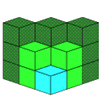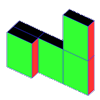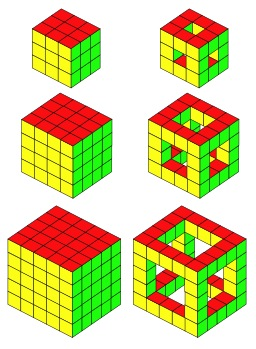#### You may also likeIf you had 36 cubes, what different cuboids could you make?### Construct-o-straws

Make a cube out of straws and have a go at this practical challenge.### Cereal Packets

How can you put five cereal packets together to make different shapes if you must put them face-to-face?

# Holes

##### Age 5 to 11Challenge LevelHere we have three solid cubes and three cubes that have holes.
They're just the first three in a series that could go on and on.

I was wondering about the number of cubes used in each ...

Then I thought about the difference between those numbers.

So, for example, I found that the first cube, $3$ by $3$ by $3$, used $27$ cubes.

The same cube with holes used $20$ cubes, a difference of $7$.

You can call these types of cubes what you like but I called them "Solid" and "Frame".

How about exploring the numbers for the next few Solid and Frame cubes?

Do you notice any patterns?
Can you explain any of the patterns?